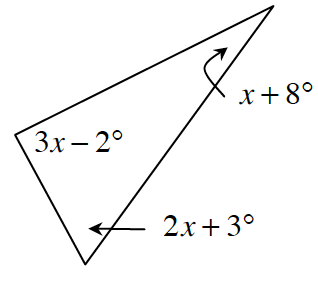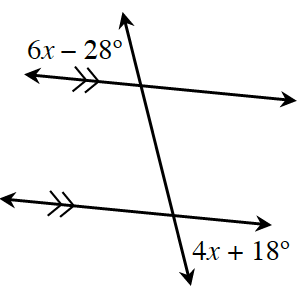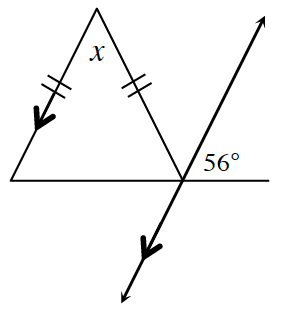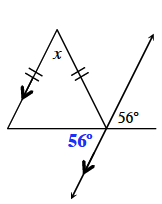### Home > GB8I > Chapter 8 Unit 9 > Lesson INT1: 8.2.3 > Problem8-122

8-122.

Use the relationships in the diagrams below to solve for $x$, if possible. If it is not possible, state how you know. If it is possible, justify your solution by stating which geometric relationships you use.

1.The angles in a triangle sum to $180º$.
So, $(3x-2º)+(2x+3º)+(x+8º)=180º$.

1.1.How can you use the parallel lines to find an angle within the triangle?